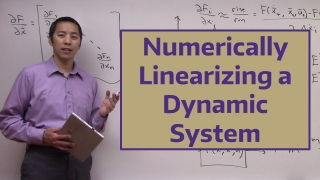# Numerically Linearizing a Dynamic System104 min
Intermediate
Video
Theory

In this video we show how to linearize a dynamic system using numerical techniques. In other words, the linearization process does not require an analytical description of the system. This is useful in situations where the system in question is either too complicated to describe with analytical functions or simply does not have an analytical representation (for example the system is a black-box that can only accept inputs and generate outputs). We review the theory of linearization before developing a numerical technique to perform the linearization. We then show how to verify our results with native Matlab/Simulink tools.

This resource is included in the following topics and journeys: